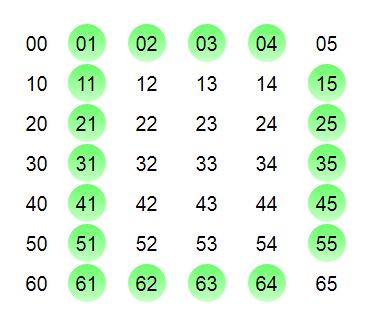# Program to print the pattern ‘D’

• Last Updated : 07 Apr, 2021

In this article, we will learn how to print the pattern D using stars and white-spaces. Given a number n, we will write a program to print the pattern D over n lines or rows.
Examples :

```Input : n = 9
Output :
******
*     *
*     *
*     *
*     *
*     *
*     *
*     *
******

Input : n = 12
Output :
*********
*        *
*        *
*        *
*        *
*        *
*        *
*        *
*        *
*        *
*        *
*********  ```

Attention reader! All those who say programming isn't for kids, just haven't met the right mentors yet. Join the  Demo Class for First Step to Coding Coursespecifically designed for students of class 8 to 12.

The students will get to learn more about the world of programming in these free classes which will definitely help them in making a wise career choice in the future.If we try to analyze this picture with a (row, column) matrix and the green circles represent the position of stars in the pattern D, we will learn the steps. Here we are performing the operations column-wise. So, for the first line of stars, we set the first if condition, where the column position with 1 gets the stars. Then all the columns greater than 1 and less than (n-2) and row position equal to 1 and n-1 get the stars. Finally, all the columns with value n-2 and row not equal to 0 or 6 get the stars, thus forming the pattern “D”. The other steps are self-explanatory and can be understood from the position of rows and columns in the diagram.
Note : Choose a minimum value of 4 for “n” to get a visible demonstration of “D” pattern.

## C++

 `// C++ program to print the pattern "D"``#include ``using` `namespace` `std;` `// Function to generate the pattern D``void` `D_Pattern(``int` `n){``    ` `    ``// loop for rows``    ``for` `(``int` `i = 0; i < n; i++){``        ` `        ``// loop for columns``        ``for` `(``int` `j = 0; j <= n; j++){``            ` `            ``// Logic to generate stars for *``            ``if` `(j == 1 || ((i == 0 || i == n-1) &&``               ``(j > 1 && j < n-2)) || (j == n-2 &&``                ``i != 0 && i != n-1))``                ``cout<< ``"*"``;``                ` `            ``// For the spaces``            ``else``                ``cout<< ``" "``;``        ``}``        ` `    ``// For changing line``    ``cout<< endl;``    ``}``}` `// Driver Code``int` `main()``{``  ``int` `n = 9;``  ` `  ``// Function calling``  ``D_Pattern(n);``  ``return` `0;``}` `// This article is contributed by mits`

## Java

 `// Java program to print the pattern "D"``import` `java.util.*;` `class` `GFG {` `    ``// Function to generate the pattern D``    ``static` `void` `D_Pattern(``int` `n){``        ` `        ``// loop for rows``        ``for` `(``int` `i = ``0``; i < n; i++){``            ` `            ``// loop for columns``            ``for` `(``int` `j = ``0``; j <= n; j++){``                ` `                ``// Logic to generate stars``                ``// for *``                ``if` `(j == ``1` `|| ((i == ``0` `||``                             ``i == n-``1``) &&``                   ``(j > ``1` `&& j < n-``2``)) ||``                   ``(j == n-``2` `&& i != ``0` `&&``                               ``i != n-``1``))``                    ``System.out.print(``"*"``);``                    ` `                ``// For the spaces``                ``else``                    ``System.out.print(``" "``);``            ``}``            ` `        ``// For changing line``        ``System.out.println();``        ``}``    ``}``    ` `    ``// Driver Code``    ``public` `static` `void` `main(String[] args)``    ``{``        ``int` `n = ``9``;` `        ``// Function calling``        ``D_Pattern(n);``    ``}``}` `// This code is contributed by ChitraNayal.`

## Python3

 `# Python program to print the pattern "D"``# Function to generate the pattern D``def` `D_Pattern(string, n):``    ` `    ``# loop for rows``    ``for` `i ``in` `range``(``0``, n):   ``        ` `        ``# loop for columns``        ``for` `j ``in` `range``(``0``, n):``            ` `            ``# Logic to generate stars for *``            ``if` `(j ``=``=` `1` `or` `((i ``=``=` `0` `or` `i ``=``=` `n``-``1``) ``and``               ``(j > ``1` `and` `j < n``-``2``)) ``or` `(j ``=``=` `n``-``2` `and``                ``i !``=` `0` `and` `i !``=` `n``-``1``)): ``                ``string ``=` `string ``+` `"*"`   `            ` `            ``# For the spaces``            ``else``:     ``                ``string ``=` `string ``+` `" "``                ` `        ``# For changing line``        ``string ``=` `string ``+` `"\n"`   `    ``return``(string);` `# Driver Code``string ``=` `"";``n ``=` `9``print``(D_Pattern(string, n));`

## C#

 `// C# program to print``// the pattern "D"``using` `System;` `class` `GFG``{``    ``// Function to generate``    ``// the pattern D``    ``static` `void` `D_Pattern(``int` `n)``    ``{``        ` `        ``// loop for rows``        ``for` `(``int` `i = 0; i < n; i++)``        ``{``            ` `            ``// loop for columns``            ``for` `(``int` `j = 0; j <= n; j++)``            ``{``                ` `                ``// Logic to generate``                ``// stars for *``                ``if` `(j == 1 || ((i == 0 ||``                           ``i == n - 1) &&``                 ``(j > 1 && j < n - 2)) ||``                 ``(j == n - 2 && i != 0 &&``                          ``i != n - 1))``                    ``Console.Write(``"*"``);``                    ` `                ``// For the spaces``                ``else``                    ``Console.Write(``" "``);``            ``}``            ` `        ``// For changing line``        ``Console.WriteLine();``        ``}``    ``}``    ` `    ``// Driver Code``    ``static` `public` `void` `Main ()``    ``{``        ``int` `n = 9;``        ` `        ``// Function calling``        ``D_Pattern(n);``    ``}``}` `// This code is contributed by ajit`

## PHP

 ` 1 ``and` `\$j` `< ``\$n``-2)) ``or` `(``\$j` `== ``\$n``-2 ``and``                ``\$i` `!= 0 ``and` `\$i` `!= ``\$n``-1))``                ``echo` `"*"``;``                ` `            ``# For the spaces``            ``else` `                ``echo` `" "``;    ``        ``}``        ` `    ``# For changing line``    ``echo` `"\n"``;``    ``}``}` `// Driver Code``\$n` `= 9;``D_Pattern(``\$n``)``?>`

## Javascript

 ``

Output :

``` ******
*     *
*     *
*     *
*     *
*     *
*     *
*     *
****** ```

My Personal Notes arrow_drop_up